# 1.什么是C语言

C语言是一门通用计算机编程语言，广泛应用于底层开发。C语言的设计目标是提供一种能以简易的方式编译、处理低级存储器、产生少量的机器码以及不需要任何运行环境支持便能运行的编程语言。尽管C语言提供了许多低级处理的功能，但仍然保持着良好跨平台的特性，以一个标准规格写出的C语言程序可在许多电脑平台上进行编译，甚至包含一些嵌入式处理器（单片机或称MCU）以及超级电脑等作业平台。

C语言是一门面向过程的计算机编程语言，与C++，Java等面向对象的编程语言有所不同。其编译器主要有Clang、GCC、WIN-TC、SUBLIME、MSVC、Turbo C等

# 2.第一个C语言程序

``````#include <stdio.h>
int main()
{

printf("hello\n");
printf("he he");
return 0;
}
//解释：
//main函数是程序的入口
//一个工程中main函数有且仅有一个
``````

\n是换行符号，执行结果如下：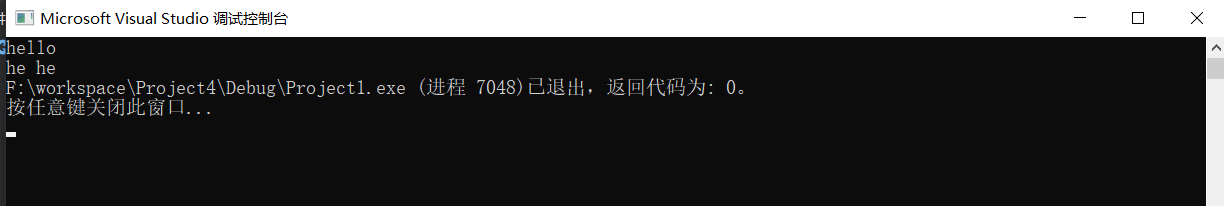# 3.数据类型

``````char        //字符数据类型
short       //短整型
int         //整形
long        //长整型
long long   //更长的整形
float       //单精度浮点数
double      //双精度浮点数
//C语言有没有字符串类型？/无
``````
• 为什么出现这么的类型？
• 每种类型的大小是多少？

``````#include <stdio.h>
int main()
{

printf("%d\n", sizeof(char));
printf("%d\n", sizeof(short));
printf("%d\n", sizeof(int));
printf("%d\n", sizeof(long));
printf("%d\n", sizeof(long long));
printf("%d\n", sizeof(float));
printf("%d\n", sizeof(double));
printf("%d\n", sizeof(long double));
return 0; }
``````

sizeof()通常用来计算一个类型或者变量的大小，单位是字节，可以看见各种类型的字节大小，运行结果如下：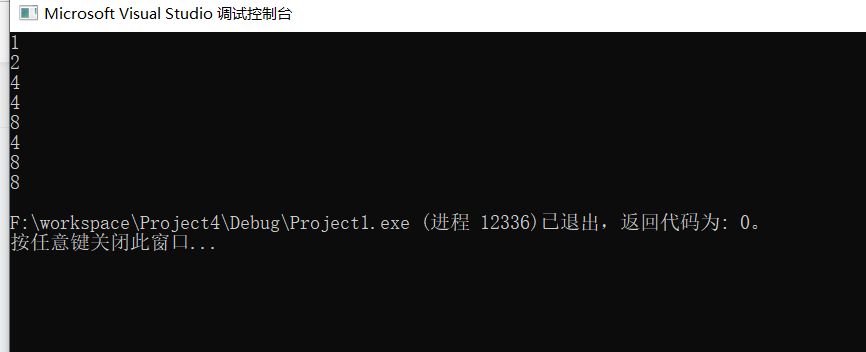``````char ch = 'w';
int weight = 120;
int salary = 20000;
``````

# 4. 变量、常量

## 4.1定义变量的方法

int age = 150;
float weight = 45.5f;
char ch = ‘w’;

## 4.2变量的分类

• 局部变量
• 全局变量
``````#include <stdio.h>
int global = 2019;//全局变量
int main()
{

int local = 2018;//局部变量
//下面定义的global会不会有问题？
int global = 2020;//局部变量
printf("global = %d\n", global);
return 0; }
``````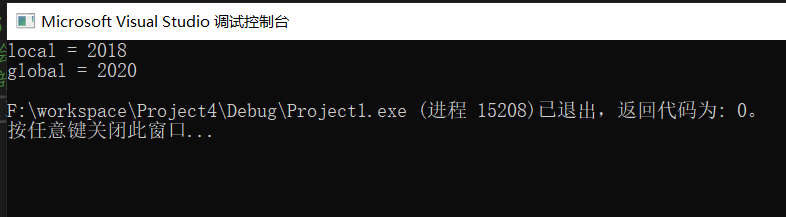## 4.3变量的使用

``````#include <stdio.h>
int main()
{

int num1 = 0;
int num2 = 0;
int sum = 0;
printf("输入两个操作数:>");
scanf("%d %d", &num1, &num2);
sum = num1 + num2;
printf("sum = %d\n", sum);
return 0; }
//这里介绍一下输入，输出语句
//scanf
//printf
``````

## 4.4变量的作用域及生命周期

• 局部变量的作用域是变量所在的局部范围。
• 全局变量的作用域是整个工程。

• 局部变量的生命周期是：进入作用域生命周期开始，出作用域生命周期结束。
• 全局变量的生命周期是：整个程序的生命周期。

## 4.5常量

C语言中的常量和变量的定义的形式有所差异。
C语言中的常量分为以下以下几种：

• 字面常量
• const 修饰的常变量
• const 修饰的常变量
• #define 定义的标识符常量
• 枚举常量
``````#include <stdio.h>
//举例
enum Sex
{

MALE,
FEMALE,
SECRET
};
//括号中的MALE,FEMALE,SECRET是枚举常量
int main()
{

3.14;//字面常量
1000;//字面常量
const float pai = 3.14f;   //const 修饰的常量
pai = 5.14;//ok?
#define MAX 100            //#define的标识符常量
return 0; }
``````

const int n=10;
n=20;//不能修改其值
int arr [n]={0};//n虽然被const修饰，但其本质上仍然是一个变量，不能用来指定数组的大小

define定义的标识符常量，不能改变其值，并且可以作为数组的大小

# 5.字符串+转义字符+注释

## 5.1字符串

C语言没有字符串类型
“hello bit.\n”

``````#include <stdio.h>
//下面代码，打印结果是什么？为什么？（突出'\0'的重要性）
int main()
{

char arr1[] = "bit";//在字符串末尾隐藏一个\0,是字符串的结束表中
char arr2[] = {

'b', 'i', 't'};
char arr3[] = {

'b', 'i', 't', '\0'};
printf("%s\n", arr1);
printf("%s\n", arr2);
printf("%s\n", arr3);//与arr1打印结果一样，\0是字符串的结束标志
return 0; }
``````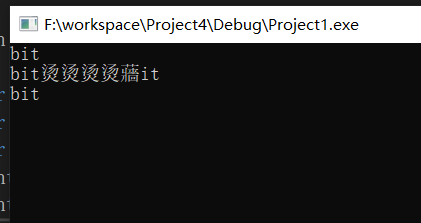int len1 = strlen(arr1);
int len2 = strlen(arr2);
int len3 = strlen(arr3);

``````printf("len1 = %d\n", len1);
printf("len2 = %d\n", len2);
printf("len3 = %d\n", len3);
``````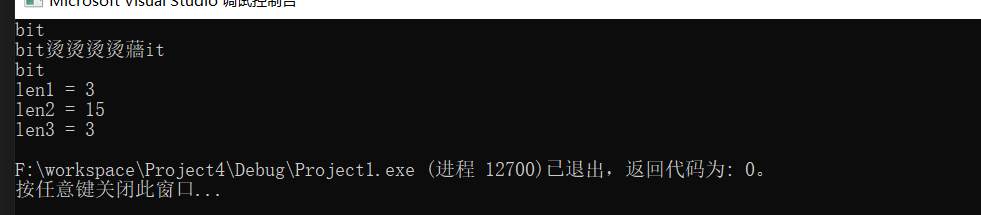## 5.2转义字符

``````#include <stdio.h>
int main()
{

printf("c:\code\test.c\n");
return 0; }
``````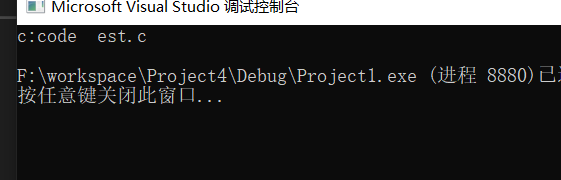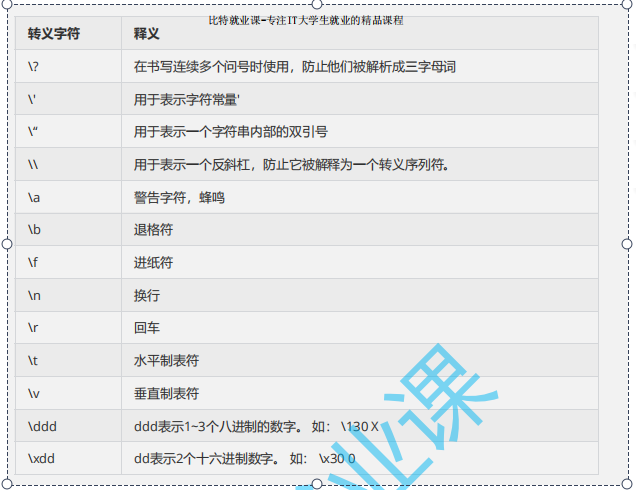• 问题1：在屏幕上打印一个单引号’，怎么做？
• 问题2：在屏幕上打印一个字符串，字符串的内容是一双引号“
``````#include <stdio.h>
int main()
{

//问题1：在屏幕上打印一个单引号'，怎么做？
//问题2：在屏幕上打印一个字符串，字符串的内容是一个双引号“，怎么做？
printf("%c\n", '\'');//打印字符
printf("%s\n", "\"");//打印字符串
return 0; }
``````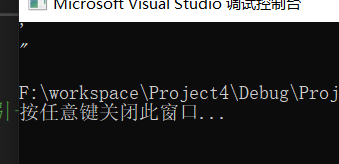``````//程序输出什么？
#include <stdio.h>
int main()
{

printf("%d\n", strlen("abcdef"));//6
// \32被解析成一个转义字符
printf("%d\n", strlen("c:\test\328\test.c"));//14
return 0; }
``````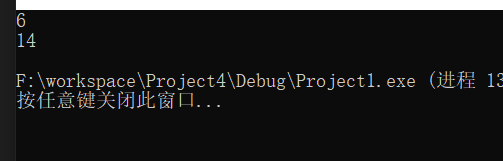``````#include <stdio.h>
int main()
{

printf("c:\\code\\test.c\n");
return 0; }
``````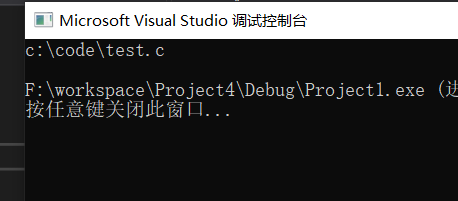\ddd转义字符
ddd表示1-3个八进制的数字，如：\130X

``````#include <stdio.h>
int main()
{

printf("%c\n",'\162');
return 0;
}
``````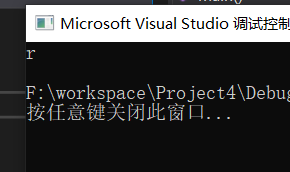ASCII码表：ASCII码表

***\xdd转义字符表示2个十六进制，如：\x30 ***

``````#include <stdio.h>
int main()
{

printf("%c\n",'\x62');//b
return 0;
}
``````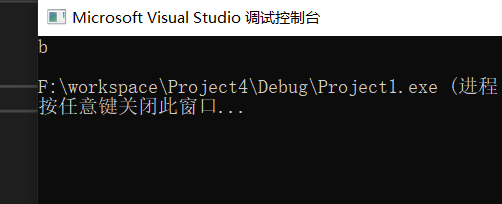# 6.注释

• 代码中有不需要的代码可以直接删除，也可以注释掉
• 代码中有些代码比较难懂，可以加一下注释文字
比如：
``````#include <stdio.h>
int Add(int x, int y) {

return x+y; }
/*C语言风格注释
int Sub(int x, int y)
{
return x-y;
}
*/
int main()
{

//C++注释风格
//int a = 10;
return 0; }
``````

***注释有两种风格：

• C语言风格的注释 /xxxxxx/
缺陷：不能嵌套注释
• C++风格的注释 //xxxxxxxx
可以注释一行也可以注释多行***

# 7.选择语句

``````#include <stdio.h>
int main()
{

int coding = 0;
printf("你会去敲代码吗？（选择1 or 0）:>");
scanf("%d", &coding);
if(coding == 1)
{

prinf("坚持，你会有好offer\n");
}
else
{

printf("放弃，回家卖红薯\n");
}
return 0; }

``````

# 8.循环语句

C语言中如何实现循环呢？

• while语句-讲解
• for语句（后期讲）
• do … while语句（后期讲）
``````//while循环的实例
#include <stdio.h>
int main()
{

printf("加入比特\n");
int line = 0;
while(line<=20000)
{

line++;
printf("我要继续努力敲代码\n");
}
if(line>20000)
printf("赢取白富美\n");
return 0; }

``````

# 9.函数

``````#include <stdio.h>
int main()
{

int num1 = 0;
int num2 = 0;
int sum = 0;
printf("输入两个操作数:>");
scanf("%d %d", &a, &b);
sum = num1 + num2;
printf("sum = %d\n", sum);
return 0; }

//***上述代码，写成函数如下：***
#include <stdio.h>
int Add(int x, int y) {

int z = x+y;
return z; }
int main()
{

int num1 = 0;
int num2 = 0;
int sum = 0;
printf("输入两个操作数:>");
scanf("%d %d", &num1, &num2);
printf("sum = %d\n", sum);
return 0; }
``````

# 10.数组

C语言中给了数组的定义：一组相同类型元素的集合

## 10.1数组定义

int arr = {1,2,3,4,5,6,7,8,9,10};//定义一个整形数组，最多放10个元素

## 10.2数组的下标

int arr = {1,2,3,4,5,6,7,8,9,10}

## 10.3数组的使用

``````#include <stdio.h>
int main()
{

int i = 0;
int arr = {

1,2,3,4,5,6,7,8,9,10};
for(i=0; i<10; i++)
{

printf("%d ", arr[i]);
}
printf("\n");
return 0; }
``````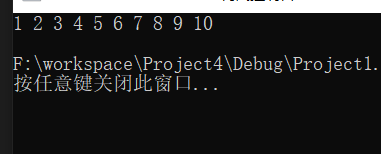# 11.操作符

``````+    -    *    /   %
``````

``````>>    <<
``````

& ^ |

= += -= *= /= &= ^= |= >>= <<=

! 逻辑反操作
- 负值
+ 正值
& 取地址
sizeof 操作数的类型长度（以字节为单位）
~ 对一个数的二进制按位取反
– 前置、后置–
++ 前置、后置++
* 间接访问操作符(解引用操作符) (类型) 强制类型转换

``````>
=
<=
<=
!=   用于测试“不相等”
==      用于测试“相等”
``````

&&
||

exp1 ？ exp2 ：exp3

exp1,exp2,exp3 ,…expN

> [] () . ->

# 12.常见的关键字

auto break case char const continue default do double else
enum extern float for goto if int long register return
short signed sizeof static struct switch typedef union unsigned
void volatile while

## 12.1关键字typedef

typedef 顾名思义是类型定义，这里应该理解为类型重命名。

``````//将unsigned int 重命名为uint_32, 所以uint_32也是一个类型名
typedef unsigned int uint_32;
int main()
{

//观察num1和num2,这两个变量的类型是一样的
unsigned int num1 = 0;
uint_32 num2 = 0;
return 0; }
``````

## 12.2关键字static

static是用来修饰变量和函数的

1. 修饰局部变量-静态局部变量
2. 修饰全局变量-静态全局变量
3. . 修饰函数-静态函数

### 12.2.1修饰局部变量

``````//代码1
#include <stdio.h>
void test()
{

int i = 0;
i++;
printf("%d ", i);
}
int main()
{

int i = 0;
for(i=0; i<10; i++)
{

test();
}
return 0; }
//代码2
#include <stdio.h>
void test()
{

//static修饰局部变量
static int i = 0;
i++;
printf("%d ", i);
}
int main()
{

int i = 0;
for(i=0; i<10; i++)
{

test();
}
return 0;}
``````

static修饰局部变量改变了变量的生命周期，让静态局部变量出了作用域依然存在，到程序结束，